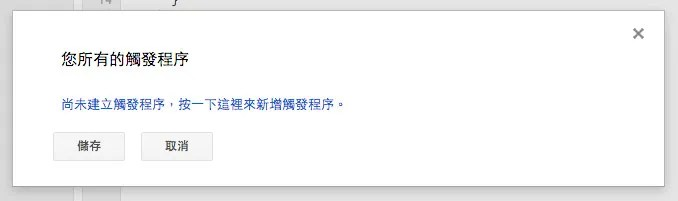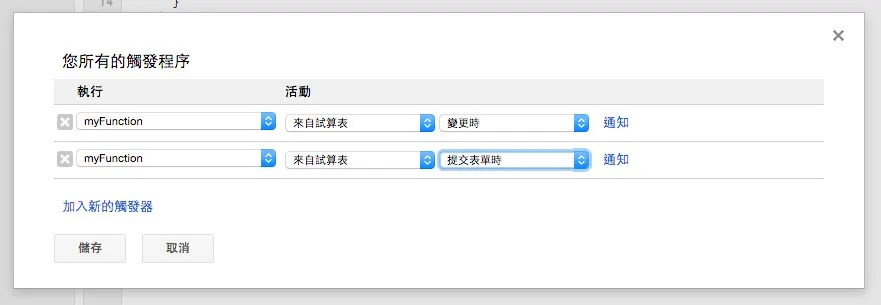# LED ( Google 表單操控 )

## 接線與實作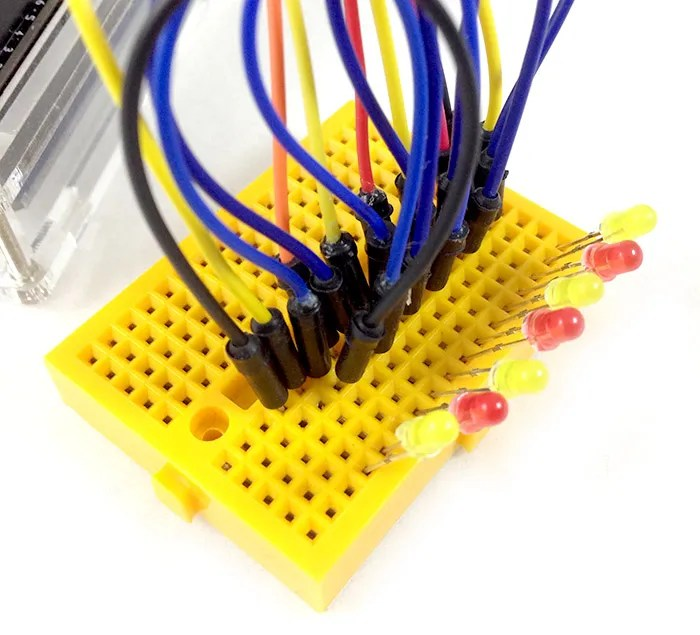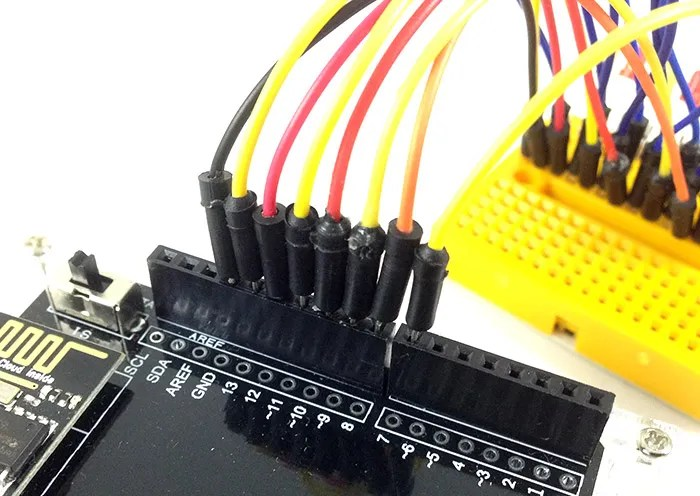## 操作解析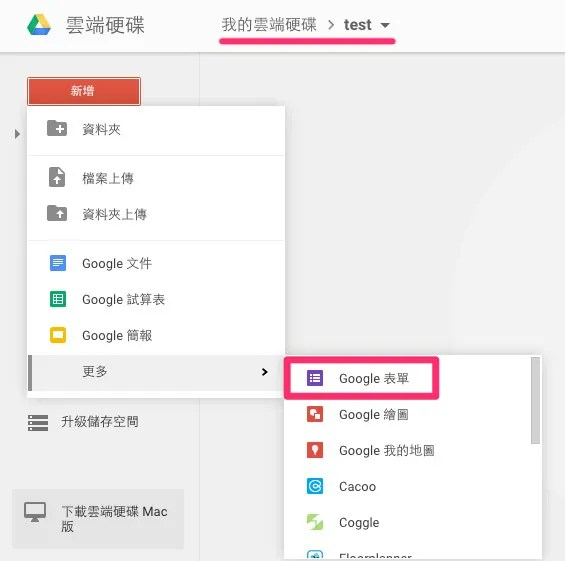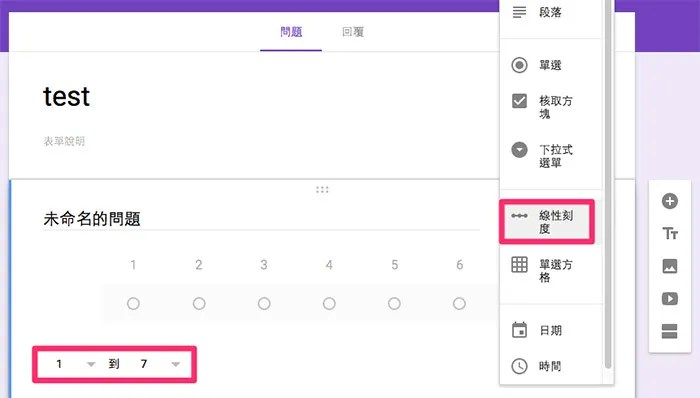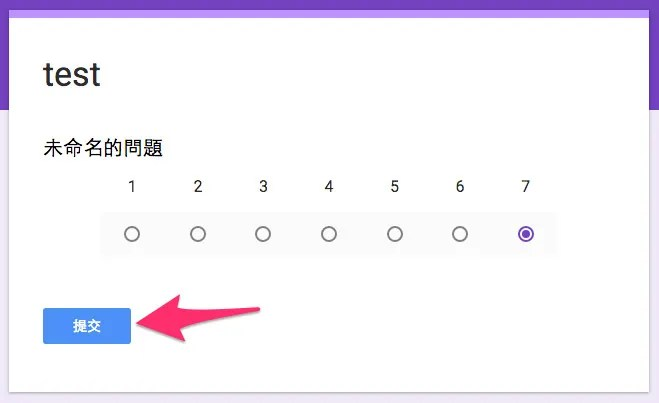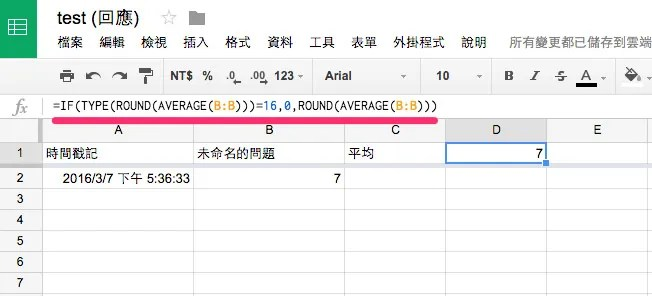``````=IF(TYPE(ROUND(AVERAGE(B:B)))=16,0,ROUND(AVERAGE(B:B)))
``````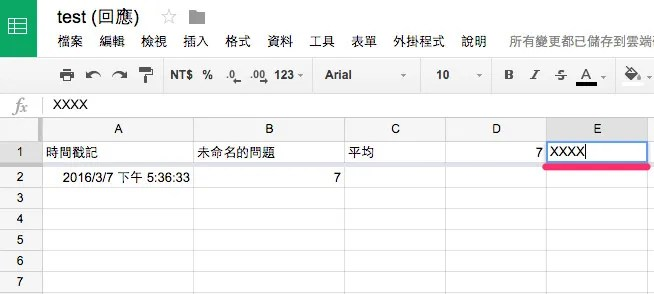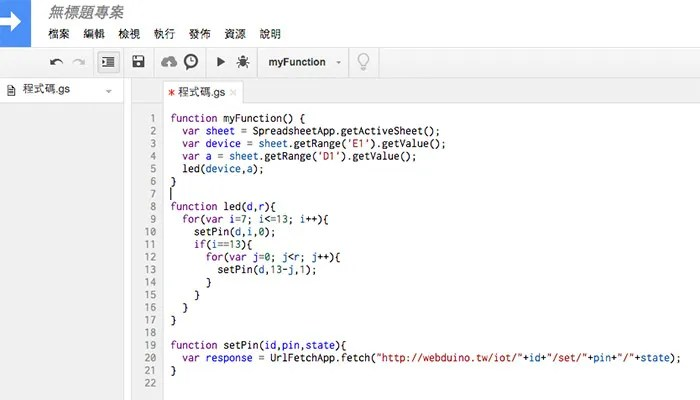``````function myFunction() {
var device = sheet.getRange('E1').getValue();
var a = sheet.getRange('D1').getValue();
led(device,a);
}

function led(d,r){
for(var i=7; i<=13; i++){
setPin(d,i,0);
if(i==13){
for(var j=0; j<r; j++){
setPin(d,13-j,1);
}
}
}
}

function setPin(id,pin,state){
var response = UrlFetchApp.fetch("http://webduino.tw/iot/"+id+"/set/"+pin+"/"+state);
}
``````